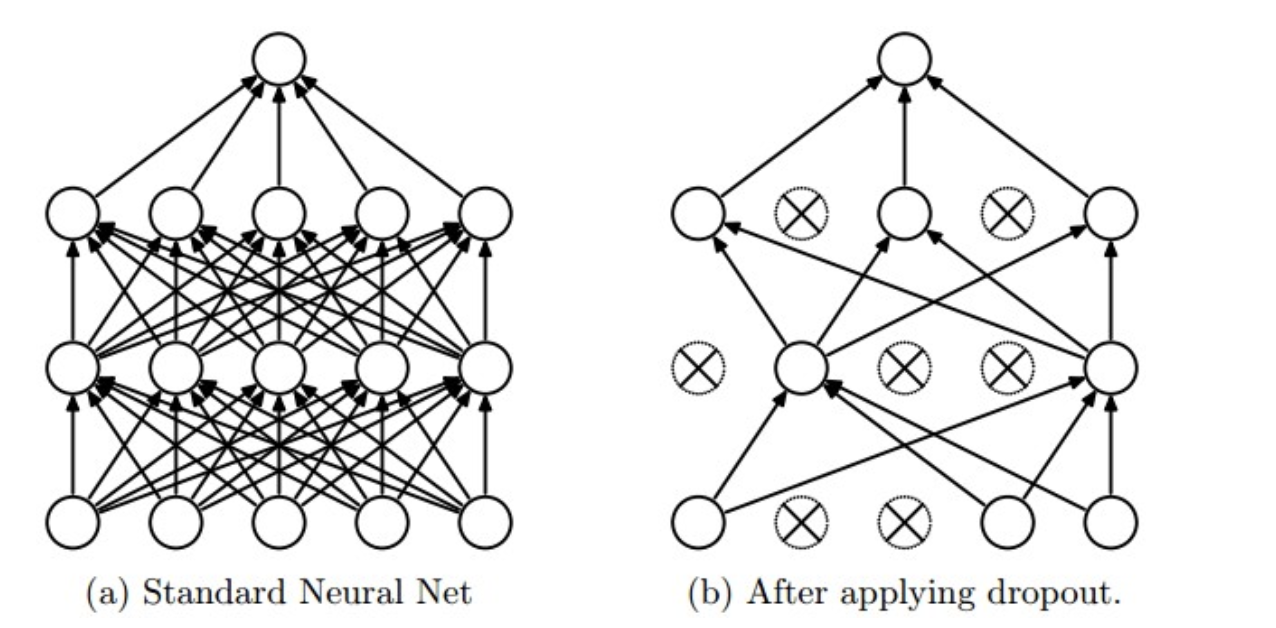# Dropout原理与实现

Dropout是深度学习中的一种防止过拟合手段，在面试中也经常会被问到，因此有必要搞懂其原理。

## 1 Dropout的运作方式

在神经网络的训练过程中，对于一次迭代中的某一层神经网络，先随机选择中的一些神经元并将其临时隐藏(丢弃)，然后再进行本次训练和优化。在下一次迭代中，继续随机隐藏一些神经元，如此直至训练结束。由于是随机丢弃，故而每一个mini-batch都在训练不同的网络。

在训练时，每个神经单元以概率$p$被保留(Dropout丢弃率为$1-p$)；在预测阶段（测试阶段），每个神经单元都是存在的，权重参数$w$要乘以$p$，输出是：$pw$。示意图如下：前一层隐藏层的一个神经元在$dropout$之前的输出是$x$，训练时$dropout$之后的期望值是$E=px+(1−p) \dot 0$; 在预测阶段该层神经元总是激活，为了保持同样的输出期望值并使下一层也得到同样的结果，需要调整$x->px$. 其中$p$是Bernoulli分布（0-1分布）中值为1的概率。

## 2 Dropout 实现

如前文所述，在训练时随机隐藏部分神经元，在预测时必须要乘上p。代码如下：

 1 import numpy as np
2
3 p = 0.5  # 神经元激活概率
4
5 def train_step(X):
6     """ X contains the data """
7
8     # 三层神经网络前向传播为例
9     H1 = np.maximum(0, np.dot(W1, X) + b1)
10     U1 = np.random.rand(*H1.shape) < p   # first dropout mask
11     H1 *= U1 # drop!
12     H2 = np.maximum(0, np.dot(W2, H1) + b2)
13     U2 = np.random.rand(*H2.shape) < p # second dropout mask
14     H2 *= U2 # drop!
15     out = np.dot(W3, H2) + b3
16
17
18 def predict(X):
19     # ensembled forward pass
20     H1 = np.maximum(0, np.dot(W1, X) + b1) * p    # NOTE: scale the activations
21     H2 = np.maximum(0, np.dot(W2, H1) + b2) * p   # NOTE: scale the activations
22     out = np.dot(W3, H2) + b3

## 3 反向Dropout

一个略有不同的方法是使用反向Dropout(Inverted Dropout)。该方法包括在训练阶段缩放激活函数，从而使得其测试阶段保持不变。比例因子是保持概率的倒数即$1/p$。所以我们换一个思路，在训练时候对数据进行1/p缩放，在训练时，就不需要做什么了。

反向Dropout只定义一次模型并且只改变了一个参数（保持/丢弃概率）以使用同一模型进行训练和测试。相反，直接Dropout，必须要在测试阶段修改网络。因为如果你不乘以比例因子p，神经网络的输出将产生更高的相对于连续神经元所期望的值（因此神经元可能饱和）：因此反向Dropout是更加常见的实现方式。代码如下：

 1 p = 0.5 # probability of keeping a unit active. higher = less dropout
2
3 def train_step(X):
4     # forward pass for example 3-layer neural network
5     H1 = np.maximum(0, np.dot(W1, X) + b1)
6     U1 = (np.random.rand(*H1.shape) < p) / p # first dropout mask. Notice /p!
7     H1 *= U1 # drop!
8     H2 = np.maximum(0, np.dot(W2, H1) + b2)
9     U2 = (np.random.rand(*H2.shape) < p) / p # second dropout mask. Notice /p!
10     H2 *= U2 # drop!
11     out = np.dot(W3, H2) + b3
12
13     # backward pass: compute gradients... (not shown)
14     # perform parameter update... (not shown)
15
16 def predict(X):
17     # ensembled forward pass
18     H1 = np.maximum(0, np.dot(W1, X) + b1) # no scaling necessary
19     H2 = np.maximum(0, np.dot(W2, H1) + b2)
20     out = np.dot(W3, H2) + b3

## 4 Dropout为什么可以防止过拟合？

1）取平均的作用

先回到标准的模型即没有dropout，我们用相同的训练数据去训练5个不同的神经网络，一般会得到5个不同的结果，此时我们可以采用 “5个结果取均值”或者“多数取胜的投票策略”去决定最终结果。例如3个网络判断结果为数字9,那么很有可能真正的结果就是数字9，其它两个网络给出了错误结果。这种“综合起来取平均”的策略通常可以有效防止过拟合问题。因为不同的网络可能产生不同的过拟合，取平均则有可能让一些“相反的”拟合互相抵消。dropout掉不同的隐藏神经元就类似在训练不同的网络，随机删掉一半隐藏神经元导致网络结构已经不同，整个dropout过程就相当于对很多个不同的神经网络取平均。而不同的网络产生不同的过拟合，一些互为“反向”的拟合相互抵消就可以达到整体上减少过拟合。

（2）减少神经元之间复杂的共适应关系